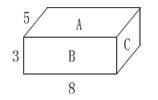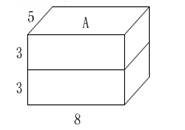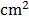### Sample Problem

In how many ways can we combine two 8 cm × 5 cm × 3 cm boxes into a big box? What is the surface area of each big box?

The surface area could be cm2 , cm2 or cm2 (list your answers in ascending order) .

#### Solution

We can combine the boxes in 3 ways: (1) one on top of the other, (2) one in front of the other, and (3) one next to the other.

First, we calculate the surface area to be 2 (5 × 3 + 5 × 8 + 3 × 8) = 158 cm2.(1) If you put one box on top of the other, we are adding the surface area of two boxes, but two faces aren’t part of the surface area. These faces have area 5 × 8 = 40. The complete surface area is 158 + 158 – 40 – 40 = 236 cm2.(2) If you put one box in front of the other, we are adding the surface area of two boxes, but two faces aren’t part of the surface area. These faces have area 3 × 8 = 24. The complete surface area is 158 + 158 – 24 – 24 = 268 cm2.(3) If you put one box next to the other, we are adding the surface area of two boxes, but two faces aren’t part of the surface area. These faces have area 3 × 5 = 15. The complete surface area is 158 + 158 - 15 - 15 = 286.